# Explore wxMaxima: Basic Operations, Equations, Calculus, Matrices, and Plotting

wxMaxima is a document based interface for the computer algebra system Maxima. wxMaxima provides menus and dialogs for many common maxima commands, autocompletion, inline plots and simple animations. Download from here.

Basic Operations

1. Using wxMaxima compute the sum of two numbers and display the result.

Code & Output: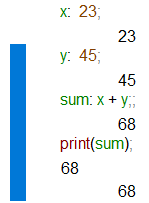2. Using wxMaxima subtract one number from another and print the output.

Code & Output: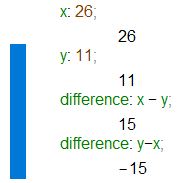3. Using wxMaxima multiply two numbers and show the product.

Code & Output: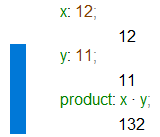4. Using wxMaxima divide one number by another and display the quotient.

Code & Output: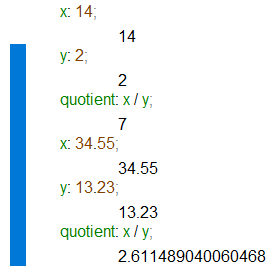5. Using wxMaxima calculate the square and cube root of a given number.

Code & Output: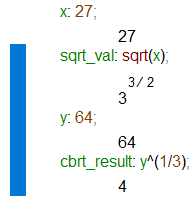6. Using wxMaxima calculate the factorial of a given number n and display the result.

Code & Output: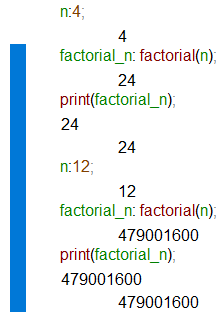7. Using wxMaxima find the maximum and minimum values among a set of numbers.

Code & Output: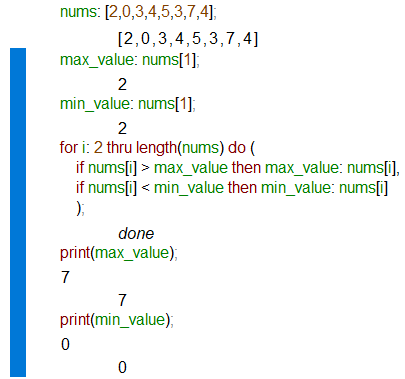8. Using wxMaxima calculate the sine, cosine, and tangent of an angle in degrees.

Code & Output: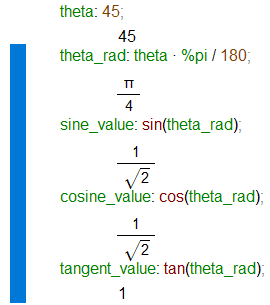9. Using wxMaxima perform matrix operations such as addition, subtraction, and multiplication.

10. Solve a system of linear equations and display the solution.

Equations and Expressions:

1. Using wxMaxima solve a linear equation of the form ax + b = c for x.

2. Using wxMaxima evaluate the value of a quadratic expression given specific values of x.

3. Using wxMaxima simplify a given algebraic expression.

4. Using wxMaxima solve a system of two linear equations with two variables.

5. Using wxMaxima evaluate the value of a trigonometric expression using predefined functions like sin, cos, or tan.

Calculus:

1. Using wxMaxima find the derivative of a given function with respect to a variable.

2. Using wxMaxima calculate the definite integral of a function over a specific interval.

3. Using wxMaxima solve a differential equation using the dsolve function.

4. Using wxMaxima determine the local extrema (minima or maxima) of a function.

5. Using wxMaxima find the limit of a function as it approaches a specific value.

Matrices and Vectors:

1. Using wxMaxima perform matrix addition and subtraction.

2. Using wxMaxima multiply a matrix by a scalar.

3. Using wxMaxima multiply two matrices together.

4. Using wxMaxima calculate the determinant of a given matrix.

5. Using wxMaxima find the eigenvectors and eigenvalues of a matrix.

Plotting and Visualization:

1. Using wxMaxima plot the graph of a given function.

2. Using wxMaxima create a scatter plot using a set of data points.

3. Using wxMaxima add labels, titles, and legends to a plot.

4. Using wxMaxima customize the appearance of a plot, such as changing the color or style.

5. Combine multiple plots into a single figure using the wxMaxima plotting functions.

﻿